Home

Newton’s Incredible Laws

Introduction.

This book discusses Newton’s laws of mechanics and gravitation in a non-mathematical way. A rudimentary familiarity with calculus will enhance the reading experience, but is not necessary. Any student contemplating an introductory college level course in mechanics will benefit from reading it. And those who have completed such a course may gain new insight into the three laws that profoundly changed the world.

The first chapter, on coordinate systems and frames of reference, sets the stage for Newton’s three laws. In Chapter 2 Newton’s first law is discussed, and the second and third laws are taken up in subsequent chapters.

Historically, Newton’s laws proved to be the well spring for several related developments (e.g. statistical mechanics, rocketry, and architecture to name but a few). Other areas of inquiry (e.g. thermodynamics and fluid dynamics), originally thought to be independent and self-contained, were in time seen also to be related to the three laws of mechanics (particularly after the discovery of atoms).

Isaac Newton announced his three laws way back in the 17th century. Years later two other great people of science, Maxwell and Einstein, laid foundations for broadening the scope of those laws and even for modifying them. Their contributions will also be discussed.

All of the statements made in this book are well-grounded in theory and experiment. Some of the field effects (discussed herein in a predominantly qualitative way) can and have been derived analytically and/or on computers. Those interested in such details are invited to visit www.grdmax.net.

Chapter 1. Coordinate Systems and Frames of Reference.

When the author was young, one of his favorite places to visit was a park where there were "monkey bars." These were a set of steel pipes forming a three-dimensional cubic grid. Many pleasant hours were spent climbing around in this grid. Although we didn't know it then, the monkey bars were our first experience with a rectangular coordinate system.

A good deal of that branch of physics called "mechanics" has to do with the study of particle behavior. For our purposes, a particle is a quantity of matter or charge concentrated practically at a point. In many cases an atom can be thought of as a particle. (Macroscopic objects … objects that we can see … can then be thought of as many atoms, bound more or less tightly to one another.) Two fundamental questions in such studies are: "Where is the particle?" and "When is it there?" Knowing the answers to these questions makes it possible to determine the particle’s velocity and acceleration at various times, and thence to ponder the "why" of such motions (as Newton did).

In order to quantitatively answer the "where" and "when" questions, we need a frame of reference. This can be defined as a coordinate system throughout which synchronized clocks are distributed.

One of the easiest coordinate systems to work with is the rectangular coordinate system (a la monkey bars). We can turn such a coordinate system into a frame of reference by putting a clock at every point where the bars intersect. Fig. 1.1 illustrates the bars in a particular layer (or plane) of a 3-dimensional rectangular coordinate system. Note that two of the rows have been labeled "x-axis" and "y-axis." Other layers (or planes) can be imagined to be laid out behind of and in front of the layer depicted in Fig. 1.1. These other planes would be equally spaced along the "z-axis," which can be imagined to be perpendicular to the page and passing through the "origin" (where x = y = z = 0).

We have constructed our coordinate system so that the distance between any two line-intersections in the x- and y-directions is one meter. But we could as well have used feet, inches, miles, light years, etc.

Figure 1.1One Plane in a Rectangular Coordinate System

If we place a clock at each point where the lines in Fig. 1.1 intersect, we have a frame of reference. As already mentioned, all of the clocks must be synchronized to be of any practical use … say they all read t = 0 at the same instant.

Table 1.1 shows data about some particular particle moving in the xy-plane. It provides partial information about where the particle was and when it was there.

Table 1.1

 Time (sec) X (meters) y (meters) 0 -3 2 1 -2 0 2 3 -1 2.5 -1 -2 3.25 0 0

Partial "Where" and "When" Data

Table 1.1 doesn’t tell us when the particle was at x = -2.5 meters, etc. We can refine our data by adding more lines in Fig. 1.1, say at half meter increments. Indeed in theory we could place lines at every value of x, y and z. Of course this strategy would only work with geometric lines (with zero thickness). We couldn’t use real bars or wires or what not. In fact we don’t usually use real wires even when the distance between the intersection points is finite (as in Fig. 1.1). The reason is obvious: real, material lines would interfere with the motion of the particle we’re observing. In physics we imagine that there is a grid (replete with clocks) through which a particle moves, and determine the where and when of the particle motion by other, less invasive means.

Now it may have occurred to you that our "where" and "when" data is somewhat subjective. We could as well have used some other instant for t = 0. And of course we could have used some other intersection point for the origin. Indeed we could have oriented our grid some other way in space (for example, with the x-axis vertical and the y-axis horizontal). The transformations from one such coordinate system to another are straightforward.

Life gets more interesting when two frames of reference move relative to one another. For example, let us suppose that a particle is at rest on the x-axis of frame A (say it is at x = 2 meters at all times). What does the particle’s motion look like relative to frame B, rotating around the z-axis (perpendicular to the page in Fig. 1.1) at a constant rate? You can probably see, in your mind’s eye, that the particle will go in a circle relative to frame B. (If frame B rotates counterclockwise around the z-axis of frame A, then the particle will travel in a clockwise circle around the z-axis of frame B.) Less obvious is the case where the origin of frame B is not at the origin of frame A (say it is at x = 1 meter) and goes in a circle around the origin of frame A. Fig. 1.2 shows the particle’s path, relative to frame B, when the respective axes of frames A and B remain parallel at all times.

Figure 1.2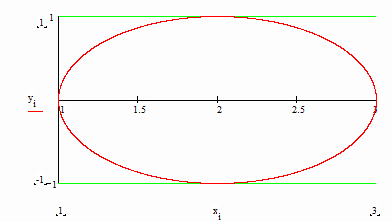Particle Path Relative to Frame B

Now one of the main questions answered by Newtonian mechanics is this: Given a force acting on a particle, what will the particle’s motion be? In Frame A the particle is at rest, and in Frame B it most decidedly is not at rest. Assuming the same force acts on the particle in both cases, which frame should we use to correlate force with particle motion? It is a fundamental issue, and Newton was evidently the first to realize its importance. Answering that question is the essence of his First Law.

Chapter 2. Newton’s First Law.

Newton’s first law answers the following question: Of the infinite set of possible reference frames, which one (or ones) should be used to correlate force with particle motion? In modern language, Newton’s first law can be stated as follows: When no force acts on a particle, then the particle remains at rest or moves with a constant velocity relative to any inertial frame of reference; inertial frames are the ones relative to which force can be correlated to particle motion.

Let us consider the particle in Chapter 1, viewed from frames A and B. If no force acted on the particle then, according to Newton, frame A would qualify as an inertial frame (since the particle remains at rest in frame A). Since the particle is not at rest in frame B, and since it doesn’t have a constant velocity in frame B (for example, its direction of motion varies in Fig. 1.2), frame B does not qualify as an inertial frame. According to Newton there is no correlation between the (zero) force acting on the particle and the particle’s motion in frame B.

In order to do the physics, Newton admonishes us to measure the "where" and "when" using the coordinate system and clocks of an inertial frame.

It should be noted that Newton does not specify any particular inertial frame. His laws hypothetically work in every inertial frame. For example, suppose (in Chapter 1) that frame C moves in the positive x-direction of frame A, at constant velocity v. If the axes of frames A and C remain parallel, then the particle moves in the negative x-direction of frame C with constant velocity –v. Newton’s first law says "at rest" or "moves with a constant velocity." So frame C is also an inertial frame. We could as well apply Newton’s second law (next chapter) in frame C, and get results in agreement with experiment.

Chapter 3. Newton’s Second Law.

Newton’s second law can be stated as follows: Relative to any inertial frame of reference, the time rate of change of a particle’s momentum equals the force acting on the particle. Particle momentum is often denoted as p. In more fundamental units, it is the product of a particle’s mass times its velocity: p=mv. Thus according to Newton, F=dp/dt=d(mv)/dt. (Newton called momentum the "quantity of motion.")

Let us suppose that a particle’s mass remains constant in time. In this case Newton’s second law can alternately be stated as follows: The force acting on a particle equals the particle’s mass times its acceleration relative to any inertial frame of reference: F=m(dv/dt)=ma. To the extent Newton’s second law works in every inertial frame of reference, we can deduce the following about "acceleration relative to inertial frames of reference": If a is the acceleration of a particle relative to one inertial frame of reference, and if all inertial observers agree on the force acting on the particle, then a is its acceleration relative to every inertial frame of reference. Case in point: Frames A and C discussed above. Presumably observers in both frames agree that zero force acts on the particle. In frame A the particle is at rest, and in frame C it moves with a constant velocity. In both cases the acceleration is zero. Indeed in any inertial frame the acceleration would be zero.

The idea, that Newton’s second law (F=ma) works in every inertial frame is referred to as co-variance. Although a particle’s velocity may differ from inertial frame to inertial frame, its acceleration is the same in every inertial frame. Thus this one law, F=ma, works in every inertial frame. It’s an amazingly beautiful and useful result.

For example, we might consider the motion of a planet (say the Earth) around the much more massive Sun (which is practically at rest in an inertial frame). Under the influence of the Sun’s gravitational force, the Earth travels nearly in a circle around the Sun once every year. Given a knowledge of what the gravitational force is, this orbit can be derived using F=ma.

But what is the situation viewed from the inertial rest frame of a space traveler, traveling past the Sun at a constant velocity? Relative to the space traveler’s frame the Sun moves in the opposite direction with a constant velocity. More importantly, the Earth’s path is not a closed orbit. It is a curve known as a cycloid. The cycloid’s shape depends upon the speed of the space traveler’s rest frame relative to the Sun’s rest frame. For every speed, however, F=ma produces the right curve! Newton’s second law is indeed co-variant!

Chapter 4. Newton’s Third Law.

In modern terms Newton’s third law can be stated as follows: Whenever some agent exerts a force on a particle, the particle exerts an equal, oppositely directed force on the agent. We have all experienced this. Whenever we push against an object, we can feel the object push back against us.

We can experimentally see that this is the case by attaching one end of a spring scale to a particle and pulling on the scale’s other end with a force F. Assuming the scale’s mass is negligible, the particle will accelerate in the direction of the applied force according to F=ma. We can tell how great the force acting on the particle is by reading the scale. But we could as easily say that the scale indicates the magnitude of the force acting on us! And that magnitude is, of course, the same as the magnitude of the force acting on the particle, the difference being that these two forces act in opposite directions.

According to Newton, things always work out this way. For every force exerted by some agent on a particle, the particle exerts an equal and oppositely directed force on the agent. Newton was the first to appreciate this fact. And as we’ll see in the next chapter, his third law contains the seeds of two great "conservation" laws.

Chapter 5. Momentum and Energy Conservation.

If we abbreviate a particle’s momentum (mv) as p, then Newton’s second law can be stated as: F=dp/dt. (dp is the infinitesimal change in momentum that occurs in the infinitesimal time interval dt. The ratio of two infinitesimal quantities can be a perfectly finite quantity. It can even be a very large quantity.) Multiplying through by dt, we find that F(dt)=dp. In words, the (infinitesimal) change of a particle’s momentum equals the product of the force acting on the particle times the (infinitesimal) time interval during which said force acts.

Now according to Newton’s third law, for all of the time a driving agent exerts the force F on a particle, the particle exerts a force F on the agent. Thus for every change of particle momentum, dp, there is a change of –dp in the agent’s momentum. The total change in momentum, of both agent and particle, is zero. In other words, the total momentum of both agent and particle is constant in time. This result … a necessary consequence of Newton’s third law … is called The Conservation of Momentum Law. It is enormously useful in solving many problems. No violation of this law has ever been observed, even when the modifications suggested by Einstein (and by experiment) are factored into Newtonian mechanics.

When we multiply the force on a particle by the distance through which the force acts, we obtain the work done on the particle. For example, when F acts in the positive x-direction (and when the particle moves in the positive x-direction), then F(dx)=dE, where dE is the infinitesimal work done by F acting through the infinitesimal distance dx. Here again, Newton’s third law implies something. For the work done on the agent, by the equal and oppositely directed force exerted by the particle, is –F(dx)=-dE. Thus the total work done on the particle and on the agent is zero.

Now work is one form of energy. If the total energy of an agent and a particle has some value before they interact, then this total energy will be unchanged by their interaction. This second consequence of Newton’s third law is called The Conservation of Energy Law. Like the Conservation of Momentum Law, it is tremendously useful in problem solving and predicting experimental results. And as in the case of momentum conservation, no violation of energy conservation has ever been detected.

Chapter 6. Force Laws.

Given knowledge of some particle’s initial position and velocity at time t=0, Newton’s second law (F=ma) can be used to calculate or compute the particle’s future motion, provided we know what F is at times greater than t=0. The question is: How do we know what F is? It is the force laws that hypothetically answer this question.

In general the force experienced by a particle can be attributed to its environment (other particles, etc.) One of the first force laws was deduced by Newton. It is usually referred to as the Law of Universal Gravitation. According to this law, every pair of particles attract one another. The force of attraction … the gravitational force … is proportional to the product of the particle masses, and inversely proportional to the square of the distance between them: F=Gm1m2/r2. (G, the proportionality constant, is referred to as the gravitational constant.)

Knowing the force law for gravity, we can plug it into Newton’s second law to get the equation of motion for mass m1, under the influence of m2: Gm1m2/r2=m1a. Indeed it was by applying his second law to the observed motions of the Moon around the Earth and the planets around the Sun (as carefully recorded by Brahe and Kepler) that Newton deduced the gravitational force law.

Another important force law was announced by Newton’s contemporary, Hooke. It states that when an agent stretches or compresses a spring, then the force exerted by the spring (on the agent and whatever anchors the spring’s other end) is proportional to the amount of stretch or compression.

In the 19th century Maxwell published the four famous equations that bear his name. These equations specify how the electric and magnetic fields of electric charge vary in space and time. The idea was that any electric charge is associated with an electric field in the surrounding space. And if the charge moves, then there may also be a magnetic field. The importance of these fields is that the electromagnetic force, experienced by some second charge, depends on the fields of the first charge at the second charge’s position.

Lorentz was the first to specify the force law for the electromagnetic force. According to Lorentz, the electric force experienced by charge qB, in the electric field of charge qA, is just F=qBE, where E is the electric field of qA at qB’s position. Maxwell’s equations prescribe how to calculate the fields of qA throughout space. Thus Maxwell and Lorentz together define one of the most important force laws in physics.

Chapter 7. Maxwell and Newton’s Third Law.

In Newtonian mechanics it is axiomatic that a particle cannot exert a force on itself. It can only exert a force on other entities (i.e. on other particles, on an agent, etc.) For many years the equal and oppositely directed reaction force, described by Newton’s third law, was something of a mystery.

Now according to Maxwell’s equations, whenever a positive charge is accelerated (relative to an inertial frame of reference) an electric field component is induced opposite to the acceleration. This induced electric field is in addition to the omnipresent divergent field that points away from any positive charge. The induced electric field is a consequence of how the magnetic field varies in time. Fig. 7.1 illustrates.

Figure 7.1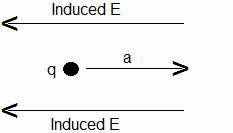Induced Electric Field, Accelerated Charge

It turns out that the induced electric field illustrated in Fig. 7.1 exists right at the charge! The Lorentz force law, F=qE, thus indicates that the charge experiences an electric force oppositely directed to a whenever a is unequal to zero. At first glance we might conclude that the Newtonian axiom … that no particle can exert a force on itself … is violated by electric charge. But it should be noted that the acceleration-opposing Lorentz force is present only when a is nonzero, and a is nonzero only when the electric charge experiences an external force (exerted by one agent or another).

Without getting into the details, it turns out that a charge can be assigned a so-called electromagnetic mass, say mem. And the acceleration, caused by an external, acceleration-producing force, is very nearly specified by F=mema. The equality is not precise in the case of charge, since the Lorentz force implied by Fig. 7.1 has two parts: an "inertial" part and a "radiation reaction" part. The inertial part is the part that equals –mema. The second part of the Lorentz force, implied by Fig. 7.1, is called the radiation reaction force. When forced to move in certain ways (e.g. when forced to oscillate back and forth on the x-axis), charges emit radiant energy in the form of electromagnetic waves. Part of the work done by the driving agent force during any given cycle is radiated away into infinite space. But here again the fields exert an equal and oppositely directed force on the driving agent. In brief, Newton’s third law (like the conservation laws) appears always to be true.

In a sense the charge is the point of interaction between the agent causing the charge to accelerate and the charge’s electromagnetic field. In cases where an agent causes a charge to accelerate, we might rephrase Newton’s third law to say: Whenever an agent exerts a force on a charge’s electromagnetic field, the field exerts an equal and oppositely directed force on the agent, the charge being the point of interaction.

Chapter 8. Charge and Neutral Matter.

Knowledge of electric charge was non-existent (or at best only very sketchy) during Newton’s time. The world view in those days was that space is populated with quantities of "neutral matter." The connection between matter and light (or electromagnetic radiation) was unknown (although Newton performed several experiments on light).

The reason why charge lay undiscovered at first is of course because atoms contain equal amounts of positive and negative charge. Today we know that "neutral matter" … even elementary particles such as neutrons … ultimately contain charged constituents (called quarks). But in Newton’s time such internal details were unknown.

When scientists discovered the existence of charge and began to experiment with it, a startling parallel between mass-mass and charge-charge interactions was noted. According to Newton’s Law of Universal Gravitation, the (attractive) force between masses m1 and m2 is Gm1m2/r2 (r being the separation of m1 and m2). And according to Coulomb’s law for charges q1 and q2, the interactive force is Kq1q2/r2 (K being another proportionality constant). The difference, in the case of charge, is that there are two varieties of charge: positive and negative. And like-signed charges repel, whereas oppositely-signed charges attract.

Since charge satisfies F=mema for many practical purposes, and (thanks to the acceleration-induced electric field) since charge always exerts an equal and oppositely directed force to any external agent force, it makes one wonder whether "neutral matter" really exists. All material particles … even uncharged ones like neutrons … contain electrically charged constituents (or quarks). As with atoms, the fields "outside" such neutral particles may cancel out more or less completely. But "inside", the individual quarks may experience the acceleration-induced electric fields discussed in Chapter 7.

There is certainly plenty to think about. The reason for bringing Maxwell and Lorentz into the Newtonian paradigm has been two-fold. First it is interesting to see that the mysterious reaction force of Newton’s third law is, at least in the case of charge, not so mysterious after all; it is an electric force, transmitted (via the charge) to an acceleration-producing agent. Secondly, the possibility that there may not, in the final analysis, be any such thing as "neutral matter" is an intriguing idea. If we look beneath the surface of neutral atoms, neutrons, etc., we may find that all of the Newtonian mechanical effects ultimately boil down to induced electric forces prescribed by Maxwell and Lorentz!

Chapter 9. Kinetic and Potential Energy.

Any particle, in motion relative to an inertial frame of reference, can do work if it is brought to a state of rest. To the extent energy is defined to be the ability to do work, the particle has energy. This energy is called kinetic energy (after the Greek word for motion).

In the pre-relativity era the formula for kinetic energy was mv2/2. The work done by a force, to change a particle’s speed from vinitial to vfinal, was m(v2final–v2initial) / 2. (This fact is sometimes referred to as the Work-Energy Theorem.)

Sometimes it may seem that the Work-Energy Theorem fails. For example, let us suppose that we lift some particle (of mass m) to a height h above the ground. We know that we must exert a force of at least GMm/R2 through a distance h, where M is the Earth’s mass, and R is its radius. (We must also exert the same force downward on the Earth. But owing to its great mass, the Earth practically remains at rest, and this downward force does negligible work.) Suppose that the particle’s speed remains constant during the lifting operation. Then its kinetic energy remains unchanged. Where did the work we expended go?

The answer lies in the concept of potential energy. The Earth-particle system’s potential energy increases in the amount mgh (where "g" is an abbreviation for GM/R2). In general we should expand the Work-Energy Theorem to state that the work done by two or more forces, exerted on a system of two or more particles, equals the change of all forms of energy.

Like kinetic energy, potential energy can do work. For example, we need only return the particle, discussed above, back to ground level. In this case, if the speed is again kept constant, the particle does work on us.

There are other forms of potential energy. For example, the potential energy of two particles, attached to the ends of a spring which is compressed or stretched an amount x, is kx2/2, where k is the so-called spring constant.

When one of the particles, in a system possessing potential energy, remains at rest in an inertial frame (for example, if one end of the spring discussed above is anchored to an immovable wall), then it is customary to attribute the potential energy to the particle that moves.

Chapter 10. Conservative Systems.

Let us suppose that, instead of lifting the particle (in Chap. 9) to a height h above the ground, we shoot it upward. If we give it the right initial speed, the particle will rise to a height h, and will then fall back to the ground. On the way up, the particle’s speed (and thus its kinetic energy) steadily decreases. And of course its potential energy steadily increases.

An important fact (and a very useful one) is that the total energy … the sum of the kinetic and potential energies … remains constant. On the way up, kinetic energy constantly converts into potential energy. And on the way down the trend is reversed. Neglecting the effects of air resistance, the particle’s speed at any particular height is the same on the way down as it was on the way up.

In going from point A to point B the particle may, in one instance, travel in a straight line (the shortest distance). Another time the particle may travel a more circuitous, longer route. But in the particle/Earth system the difference in potential energy between any two points depends only upon the relative positions of the two points, and not on the path to go from one to the other.

Such systems are said to be conservative. The hallmark is that the total energy is a system constant. That is, the sum of the kinetic and potential energy is constant.

Recalling the case (in Chap. 9), where the particle’s speed remained constant as we lifted it to height h, that system was not conservative. The kinetic energy did not change, but the potential energy increased. The case where the particle is shot up into the air, on the other hand, is conservative. In this case the sum of T (kinetic energy) plus U (potential energy) is always the same: T+U=E, where E, the total energy, is a system constant. This singular property of conservative systems is of great utility in solving many problems.

Chapter 11. Least Action.

There is a principle in optics (called Fermat’s Principle) which deals with the fact that light travels at different speeds through different media. For example, the speed of light in air is greater than it is in water. Consequently the light reflected from a fish in the water may not travel in a straight line to the fisherman’s eye (assumed to be in the air). At the air/water interface the light may abruptly change its direction.

Fermat’s Principle, or the Principle of Least Time, provides information about the light’s path. According to this principle, the path taken by the light, in going from point A to point B, is the path that requires the least time.

In mechanics there is a similar principle, dealing with a conservative system particle’s path from point A to point B, in some time interval t=(tfinal-tinitial). According to this principle, the path is such that the integral of (T-U)dt, from tinitial to tfinal, is least for the actual path taken. (T is Kinetic Energy, and U is Potential Energy.) Any other imagined path will produce a greater integral. This integral is called the "action," and the principle is called the Principle of Least Action.

Now since Newton’s second law provides a basis for computing the exact particle path in any given case, it might be wondered why we should concern ourselves with paths that never occur. The relevant fact in this matter is that computers were not available to our forebears. The abiding idea before computers was to analytically predict a particle’s motion. And there are some cases where Newton’s second law does not serve well (if at all) in such analyses. But there is an alternative, called Lagrangian mechanics, which helps fill this shortfall. And Lagrangian mechanics exploits the Principle of Least Action.

In both Newtonian and Lagrangian mechanics the particle path in any given case is perfectly precise. But in quantum mechanics it might be said that nothing is precise! The best we can say, for any given instance of particle motion, is that the particle leaves the vicinity of point A at approximately time tinitial, etc. In the case of "macroscopic" particles (or in cases of the centers of mass of macroscopic objects), the deviations from points A, B, and the geometric line predicted by Newton/Lagrange, are too small to detect! So why worry about them? The answer is: Don’t. The times when such deviations become a matter of concern is when the particles are microscopic, which is to say when their masses are on the order of an electron’s mass. In those cases the paths predicted by Newton and Lagrange (give or take a little) are only the most probable. But they aren’t the only observed paths! Indeed it is found that some of the particles, in a given "set of runs," will diverge quite significantly from the classically predicted paths. The same is true of light. That is the reality. It is the more complete picture of how the world works.

In the microscopic world, the apparent exactness and repeatability of Newton, Lagrange and Fermat opens out into a more blurred paradigm. And it is quantum theory that specifies how to figure the percentages in the world of the very small.

Chapter 12. Fictitious Forces.

In Chapter 2 it was emphasized that Newton’s laws work only in inertial frames of reference. For although subject to no force, a particle may accelerate when viewed from a non-inertial frame.

Having emphasized the importance of inertial frames, we now change our minds and say that physics can be done out of non-inertial frames! This feat is accomplished by introducing fictitious forces … forces that cannot be attributed to anything in a particle’s environment. Let us consider a simple example.

Imagine that we are standing on a large, round floor that can rotate about a vertical axis. (An example would be a merry-go-round without the horses.) When the rotation rate is zero, the only forces we experience are gravity (pulling downward) and the floor (pushing upward). These two forces sum to zero, and our acceleration is accordingly zero.

If the floor rotates, however, then relative to the inertial frame in which the axis of rotation is fixed, we go in a circle. At any given rotation rate our speed (i.e. our velocity magnitude) is constant. But we accelerate radially inward toward the floor’s center. (That is, the direction of our velocity changes constantly, and changes in velocity direction, like changes in velocity magnitude, imply an acceleration.)

One of the convenient things about Newton’s second law is that it works for both linear and radial acceleration. Thus the floor must exert an inward force upon us in order for the radial acceleration to occur. Indeed we can feel this force acting on us. If we are standing up, we must lean toward the floor’s center to keep from falling down.

Now quite often our inclination is to assume that our "personal" rest frame is an inertial frame. And viewed from the rotating floor’s (non-inertial) rest frame, it might seem that there are now four forces acting on us: gravity pulling downward, the floor pushing upward, a mysterious, persistent pull radially outward, and the floor pushing inward. Again all four forces sum to zero and we don’t accelerate in our rest frame.

The new force … the one that seems to pull us outward … is called a centrifugal force. (‘Centrifugal’ is Latin for ‘fleeing the center’.) For any given floor rotation rate, we find that this outward pointing centrifugal force is proportional to r, our distance from the rotation axis: Fcentrifugal=mw2r (mw2 being our mass (m) multiplied by the constant rotation rate (w) squared). The point is that we can use Newton’s second law in the rotating floor’s (non-inertial) rest frame provided we include the fictitious centrifugal force.

The centrifugal force resembles a gravitational force, in that its magnitude is proportional to our mass. It is as though a gravitational field points radially outward at all points in the non-inertial, rotating frame of reference, said field’s strength being proportional to the distance from the frame’s rotation axis.

There is a second fictitious force which we must contend with if we move radially inward or outward relative to the rotating frame. This force is called the Coriolis force and (like the magnetic force sometimes experienced by electric charge) it always acts at right angles to our velocity. Like the centrifugal force, the Coriolis force can be understood by applying Newton’s second law in an inertial frame (particularly in the inertial frame in which the floor’s axis of rotation is at rest).

Relative to an inertial frame our speed depends on our distance from the rotating floor’s center. The farther out we are from the center, the faster we go. To be precise, our speed is wr, where w is the floor’s rotation rate and r is our distance from the center.

What happens if we move away from the center? Viewed from the inertial frame, our speed increases … we accelerate tangentially. According to F=ma the floor must exert a tangential force to speed us up. If we are walking upright, we must not only lean backwards (toward the rotating floor’s center) to counterpoise the centrifugal force, but we must also lean to one side to counterpoise the new Coriolis force.

Like the centrifugal force, the Coriolis force depends on our mass. But it also depends on our distance from the center and the rate at which we walk away from the center. The faster we walk, the greater the force seems to be.

These fictitious forces … these forces that must be invoked if we wish to apply Newton’s second law in non-inertial frames … are of course nothing new. They aren’t real forces, and the physics can in theory always be understood in terms of real forces when things are viewed from an inertial frame. But sometimes it is mathematically more convenient to work out of a non-inertial frame. And it is the fictitious forces that make it possible to use Newton’s laws in these non-inertial frames.

It is of historic interest that Mach was dissatisfied with the concept of fictitious forces. He suggested that the centrifugal and Coriolis forces can be thought of as real forces, attributable to "the rest of the Universe." That is, Mach suggested that if the entire universe revolved around an axis fixed in an inertial frame, then any particle at rest in that frame would experience a gravitational force radially outward. And if the particle moved it might also experience a force at right angles to its velocity. In effect Mach was saying that any frame of reference can serve as an inertial frame. It was an idea that would be further developed by Einstein in his General Theory of Relativity.

Chapter 13. Spaces.

Fig. 13.1 depicts a particle, A, in the xy-plane of a rectangular coordinate system which we’ll call "K." An arrow has been drawn from the origin to A’s location. It is customary to call this arrow the displacement of A (from the origin), and to denote it using bold underline, say R(A).

Figure 13.1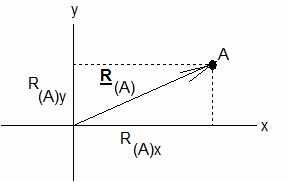Particle A in Rectangular Coordinate System K

If we drop perpendiculars from the tip of R(A) to the x- and y-axes (see the dotted lines in the figure), we get the rectangular components of R(A), say R(A)x and R(A)y. These rectangular components uniquely define the location of A in coordinate system K. Any other point will have different components. (More generally particle A might exist in 3-dimensional space, in which case its location would be uniquely defined by the three components R(A)x, R(A)y, and R(A)z.)

With regard to the displacement of A from the origin, there is nothing sacred about our coordinate system’s axes. For example, someone else might prefer to work in coordinate system K’, whose origin might coincide with the origin of K, but whose x’-axis makes an angle q with the x-axis of K, etc. In system K’ the x’-component, R(A)x’,does not equal R(A)x. But if we know what the angles between the respective axes of K and K’ are, then we need not measure the components in K’; they are precisely related to the components in K by a transformation involving the angles between the respective axes.

Quantities that transform from one coordinate system to another using this transformation are said to be vectors. And displacement is only one of many vectors. Velocity … the rate at which a displacement vector varies in time … is also a vector. And acceleration … the time rate of change of velocity … is a vector. Momentum is simply a scalar multiple of velocity, so it too is a vector. And of course Newton’s second law suggests that force … the time rate of change of momentum … is a vector.

The length of the displacement vector R(A) (in Fig. 13.1) is called the vector’s magnitude. It is often denoted in plain type (say R(A)). And it is related to the components of R(A) by the Pythagorean Theorem: R(A)2=R(A)x2+R(A)y2. (In 3 dimensions R(A)2 = R(A)x2 +R(A)y2 +R(A)z2.)

Pythagoras and other ancient Greeks (notably Euclid) worked out many important formulas in geometry (which is Greek for ‘Earth Measurement’). For example, the area of a spherical surface of radius R is 4pR2. And the volume increment between two concentric spherical surfaces, of radii R and R+dR, is 4pR2dR.

Now here is a thought provoking idea: there are as many "spaces" as there are vectors! We seem intuitively inclined to think of "displacement space" as being the one true space. But there is no reason why we shouldn’t, for example, define a velocity space. In a velocity space the "displacement" from the origin might constitute the velocity of some particle A, say v(A), with components v(A)x, v(A)y, and v(A)z. All of the rules that apply in displacement space also hold in velocity space. For example, v(A)2 = v(A)x2 + v(A)y2 + v(A)z2.

Sometimes changing from "regular" (or displacement) space to some other space will simplify a problem’s solution. A first course in mechanics may not require that students solve problems in spaces other than displacement space. Nevertheless it is a good idea to be aware that there are (mathematically speaking) as many spaces as there are vectors. Deeper insight can often be gained by thinking about things in the context of a space other than displacement space.

Chapter 14. An Example in Velocity Space.

Note: This chapter can be skipped by the beginning student. It is included to demonstrate how working in a "space" other than the usual one can lead to new insights.

Following is a derivation of g(v)dv, the Maxwell/Boltzmann distribution of molecular speeds (i.e. velocity magnitudes) in a gas containing N molecules, where N is arbitrarily large and where each molecule’s velocity is unique (so that there are N distinct velocities). As a matter of definition, g(v)dv is the fraction of the N molecules with speeds in the range v to v+dv. Maxwell derived the result in 1859. (Unlike the other chapters in this book, this chapter is necessarily more mathematical. It is offered as an example of how useful spaces other than displacement space can sometimes be.)

~

In the following discussion, "(v,dv)" is shorthand for "the range v to v+dv."

Let Ng(v)dv be the number of molecular velocities with magnitudes in (v,dv). Then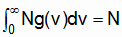. (1)

From thermodynamics, the mean kinetic energy equals 3kT/2, where k is Boltzmann’s constant and T is the absolute temperature. Thus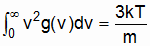. (2)

In postulating a form for g(v)dv, we expect few (or no) molecules to be standing still, and none to be moving with infinite speed. And of course g(v)dv must integrate (normalize) to unity. Integrals of the form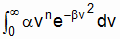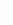have the potential of satisfying both of these requirements, provided n>0. Thus we postulate that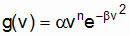, (3)

where a, b and n are constants to be determined.

Let the fraction of velocities with x-components in (vx,dvx) be f(vx)dvx. Similarly for (vy,dvy) and (vz,dvz). Then the fraction with components in (vx,dvx) and (vy,dvy) and (vz,dvz) is f(vx)f(vy)f(vz)dvxdvydvz. And the number of velocities is Nf(vx)f(vy)f(vz)dvxdvydvz.

In velocity space each velocity can be represented as a point (i.e. as the tip of a "displacement" vector from the origin). Since dvxdvydvz is a volume element in velocity space, the density of the velocity vectors at point (vx,vy,vz) is Nf(vx)f(vy)f(vz). This density can only depend on v, the "distance" from the origin.

The number of velocities in the spherical shell, of volume 4pv2dv is Ng(v)dv, and the density in this shell is Ng(v)dv/(4pv2dv). Thus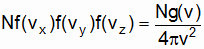(4)

In particular, for vx=v and vy= vz=0.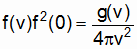, (5)

or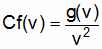(6)

where the constant C equals 4pf2(0). Now this equation can be satisfied for all v only if g(v) contains a v2 term. Thus the correct choice for n in Eq. 3 is ‘2’, and we postulate that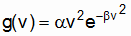. (7)

From Eq. 1,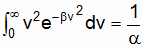. (8)

Or, since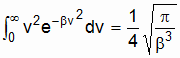, (9)

we find that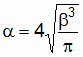(10)

and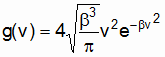. (11)

From Eq. 2 we have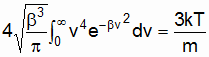. (12)

Or, since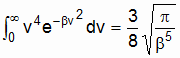, (13)

we find that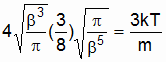(14)

and thus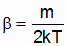. (15)

Substituting in Eq. 10,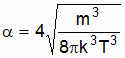, (16)

and thus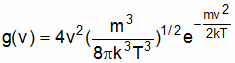. (17)

Or,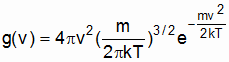, (18)

which is the Maxwell-Boltzmann distribution.

~

Maxwell’s formula for g(v)dv was experimentally corroborated by Stern in 1926. Several other experiments provided further corroboration. In brief, Maxwell was right. Other derivations by Boltzmann and Gibbs arrived at the same result.

Chapter 15. Speed-Dependent Mass.

For a long time after Newton announced his three laws of mechanics, it was believed that the mass of a particle is invariant (or constant). The Conservation Laws for momentum and energy were augmented by a Conservation of Mass Law. Given some total mass contained in a volume of space, it was believed that the mass remained constant (so long as none came into the volume from the outside, and none left the volume). This law appeared to be well-corroborated by experiment. One could burn the material, put it through diverse chemical reactions, beat it out of (or into) shape, etc. Nothing seemed to change the total mass within an experimental volume. As previously mentioned, this meant that Newton’s second law … originally written F=d(mv)/dt … could alternately be stated as F=m(dv/dt)=ma.

When physicists began thinking about the inertia of charged particles (i.e., when they began thinking about electromagnetic mass), it became clear that electromagnetic mass is not invariant; it increases as a charge’s speed increases. And when Einstein pieced together his Special Theory of Relativity it was noted that, in order for momentum to be conserved in elastic collisions between uncharged particles, the mass of these particles too would have to depend on particle speed. The equation in both the neutral mass and electromagnetic mass cases turned out to be m=mo/(1-v2/c2)1/2. "mo" in this relationship is the particle’s mass when it is at rest, and "c" is the speed of light.

The suggested dependence of mass on speed was soon corroborated by experiments with high-speed particles. The reason why this dependence remained undiscovered for so long owes to the very great speed of light. In the early days experiments were limited to cases where v was much less than c. And the adjustment term (1-v2/c2)1/2 is practically unity in such cases. But (1-v2/c2)1/2 approaches zero as v approaches c. And thus m=mo/(1-v2/c2)1/2 increases without bound as v approaches c. Among other things, it is impossible to accelerate any material or charged particle all the way up to the speed of light!

Now one of the truly wondrous things about Newton’s second law is this: despite the dependence of mass on speed, Newton’s second law is still true in the form he originally wrote it down. That is, F=d(mv)/dt is true in relativity! Newton of course couldn’t have known that mass increases without bound as v approaches c. He couldn’t have known that F=d(mv)/dt is generally true, whereas F=ma is not. (Could he???)

Chapter 16. Mass-Energy Equivalence

Albert Einstein, the author of Special (and later of General) Relativity, reasoned that mass was not only speed-dependent but, under certain circumstances, mass could be changed completely into energy (notably radiant energy). Before Einstein there were (in the minds of men) energy and matter (or mass). Each of these existed in its own world, so to speak, and the manner whereby matter emitted radiant energy was something of a mystery. Certainly, even with the dependence of mass on speed factored in, it wasn’t at first clear that mass could be completely transformed into energy (and vice versa). When Einstein suggested that this could be the case, there was understandably skepticism. Part of this skepticism no doubt stemmed from the now-famous equation, E=mc2. As already mentioned, the speed of light is great, and c2 is therefore enormous. A minuscule bit of matter could theoretically be transformed into an enormous amount of energy!

In modern times we live with the knowledge (and dread) that Einstein was right. This became common knowledge with the detonation of the first atomic bombs.

Today the controlled transformation of mass into energy is an important source of energy. When the nuclei of certain massive atoms (e.g. Uranium) are penetrated by neutrons, the nuclei "split" into various pieces … a process known as nuclear fission. The total mass of all the fission products is less than the mass of the original neutron and nucleus, and this loss of mass is manifest as energy in amounts precisely specified by E=mc2. And the whole general process works both ways. Under appropriate conditions large amounts of energy can be changed into exotic material particles!

Interestingly enough, what is true for the splitting (fission) of heavy nuclei is also true for the joining (fusion) of certain light nuclei. For example, Deuterium nuclei each contain one proton and one neutron. And when two Deuterium nuclei are fused into a Helium nucleus, energy is released. This is the process that fuels the Sun and other stars.

Technically, the problem with fusion is to get two Deuterium nuclei close enough to fuse. The repulsive forces become relatively huge when the distance between the nuclei approaches fusing range. On the Sun, this is accomplished by the immense gravitational field at the Sun’s surface (and the great speeds of particles in such a hot environment). Here in earthbound labs other techniques must be found.

The supply of Deuterium fuel in the oceans is inexhaustible. Thus solving the controlled fusion problem is one of mounting interest, as our fossil fuel reserves dwindle.

Thanks to E=mc2, the old conservation laws of mass and energy have merged into a new "super" law: the conservation of mass-energy. Provided no mass or energy crosses a volume’s boundary, the total amount of mass and energy inside the volume remains constant. But at one time there may be lots of mass and little energy; at a later time there may be less mass but more (lots more) energy!

Chapter 17. Relativistic Mechanics.

Newton’s second law, in the form F=d(mv)/dt, is relativistically correct. Many of the axiomatic beliefs and assumptions from the pre-relativity era fall, but Newton’s fabulous second law persists. (Indeed all three of his laws are relativistically correct.)

The new feature in relativity is that mass depends on particle speed. Thus (as you may know from calculus), Newton’s second law expands to F=m(dv/dt) + v(dm/dt). In general the algebra is more involved than in the good old F=ma days. But two important cases warrant mention.

When F acts perpendicular to v (for example, when a particle is made to go in a circle at constant speed), then particle mass remains constant and Newton’s second law simplifies to F=gmoa, where g is a popular shorthand for 1/(1-v2/c2)1/2. When F acts in the same (or opposite) direction as v, then Newton’s second law simplifies to F=g3moa.

There are some surprising (and even entertaining) ramifications of the dependence of mass on speed. Let us imagine that a particle accelerates at a constant rate down the y-axis of inertial frame K. A force of F=g3moa must be applied downward. (Note that F increases as v increases.)

Viewed from some second frame, K’, moving in the positive x-direction of frame K at constant speed u, the particle moves along a curved path. And, like the K observer, the K’ observer would agree that particle speed (and hence particle mass) is increasing with time.

But here is an important difference between K and K’: for the K observer the particle’s x-component of momentum is constantly zero. Thus there is no x-component of force. For the K’ observer, the particle’s x-component of velocity is constant and equal to –u. But since the K’ observer agrees that the particle’s mass is increasing, the K’ observer contends that the x’-component of momentum is steadily growing in magnitude! According to the K’ observer, there must be a negative x’-component of force!

To cut to the chase, in relativity theory all inertial observers do not agree about the force acting on a particle. In the pre-relativity era the transformation of force, from inertial frame K to inertial frame K’, was trivial: F’=F. But in relativity the transformation is more complicated. Without getting into the details, Fx’ might depend on Fx, Fy, and Fz. Similar remarks apply to the transformation of acceleration from one inertial frame to another.

The fact that the old, so-called Galilean transformation for force was (regrettably) incorrect was almost a breath of fresh air for students of electromagnetic theory. For in electromagnetism a charge might or might not have a magnetic field, depending on whether or not it was moving. And a second charge might or might not experience a magnetic force, in addition to the usual electric force.

But regarding the first charge’s magnetic field, we might wonder "moving relative to which frame?" If the charge moves with a constant velocity relative to frame K (and accordingly has a magnetic field), then it will be at rest in some other frame, K’ (and will have no magnetic field).

The answer to this conundrum is that a second charge may feel a magnetic force according to frame K, and no magnetic force according to frame K’. Classically it’s something of a paradox. But the relativistic transformation of d(mv)/dt produces precisely the same results, in frames K and K’, as are specified by Maxwell and Lorentz!

To the extent F=d(mv)/dt is relativistically correct in all inertial frames, all of the forces of physics must transform from one frame to another quite as d(mv)/dt does.

Chapter 18. The Principle of Superposition.

In chapter 12 we discussed the case where we stood on a floor that rotated around a vertical axis. Viewed from the rotation axis’ rest frame (presumed to be an inertial frame), we went in a circle. That is, we constantly accelerated radially inward toward the axis of rotation. In addition, we experienced the force of gravity. Thus the floor had to exert two forces on us: one upward and equal in magnitude to the gravitational force (since we neither accelerated upward nor downward), and one radially inward (since we did accelerate radially inward).

Now when two or more forces are exerted on a particle, they can be replaced by a single force (namely their vector sum). Replacing two or more vectors by their vector sum is generally referred to as the Principle of Superposition. For example, Fig. 18.1 depicts the single force that the floor would have to exert on Leroy (who has kindly agreed to take our place on the rotating floor), in order to make him go in a circle while remaining in the xz-plane.

Figure 18.1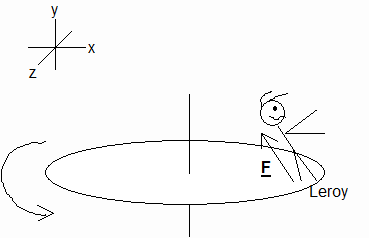F, the Combined Gravity-Counteracting and Radial Acceleration-Causing Forces.

Many problems, where a particle experiences several forces attributable to other entities in its environment, can be simplified by invoking the Principle of Superposition before applying F=ma.

It should be borne in mind that, in the case of forces, the Principle of Superposition generally applies only to particles. It may not suffice in the case of macroscopic objects, composed of many bound particles. For example, consider the case of the macroscopic bar depicted in Fig. 18.2. Equal but oppositely directed forces act on its two ends. The forces sum to zero. Yet the bar doesn’t remain motionless. Parts of it to the left of center accelerate upward, and parts to the right of center accelerate downward.

Figure 18.2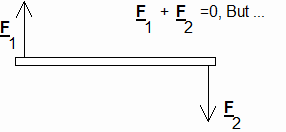When Superposition Doesn’t Suffice

As it turns out, there is one point in the bar that lends itself well to superposition. It is at the bar’s center, and it’s called the bar’s center of mass ("cm" for short). This one point has zero acceleration when all of the acting forces sum to zero. For example, in Fig. 18.2, (F1+F2)=macm=0. We shall consider the center of mass further in the next chapter.

How is it that we could use superposition in Fig. 18.1? Leroy is, after all, scarcely a particle. The reason we could use superposition in that case is because Leroy is composed of bound atoms, and the line of F (the resultant force exerted by the floor on Leroy) passes through Leroy’s cm. Leroy has learned to lean inward at just the right angle to make this be so. Were he to lean at any other angle, he would topple.

Had F1 and F2 pointed to the left and right respectively in Fig. 18.2, their mutual line of action would pass through the bar’s cm, and the whole bar’s acceleration would have been zero.

Without belaboring the point, the Principle of Superposition, in the case of multiple forces, can be useful in macroscopic, "rigid" cases as well as in particle cases, provided the lines of action of all of the forces act through the center of mass. In such cases F=ma works for all parts of the macroscopic object. That is, all parts of the object have the same motion as the object’s center of mass. (But even in these cases the individual forces may cause something to happen. For example, if equal and oppositely directed forces act to the left and right, on the ends of the bar depicted in Fig. 18.2, then the bar is stretched … the amount depending upon the bar’s elasticity. Strictly speaking, particles don’t stretch, bend, etc.)

Chapter 19. The Center of Mass.

Let us consider a "non-rigid" system of "particles," say our solar system. Relative to an inertial frame, all of the particles are accelerating. (In our solar system, even the massive Sun is not at rest in any inertial frame; it wobbles under the influence of the planets.) The Moon pulls on the Earth, the Earth pulls on Mars, etc. Every force can be accurately determined. What total force do we obtain when all of the interactive forces are summed? You can probably see, by applying Newton’s third action/reaction law, that the forces sum to zero.

Now the forces acting on a given planet are not completely specified by the attractions toward other planets and the Sun. Every "particle" in our solar system is also attracted to each and every star in our galaxy (and beyond). We can sum the forces, attracting the particles in our solar system to the nearest star, and this sum is of course not zero. In addition to accelerating toward the Sun and Mars, etc., the Earth is accelerating slightly toward the nearest star. And so is the Sun, and Mars, and Jupiter, etc. Things can get very complicated!

When we do the math, however, we find a beautifully simplifying result. There is a point, called the solar system’s center of mass, that accelerates toward the nearest star according to Newton’s second law: F(Total Force Toward Nearest Star) = m(Entire Solar System)a(Solar System’s Center of Mass). Indeed if the solar system were alone in the Universe (which seems practically to be the case, owing to the great distance to even the nearest star), then the solar system’s center of mass would be at rest in some inertial frame of reference.

Rigorously speaking, then, Newton’s second law applies to the center of mass in any given case. Sometimes the center of mass won’t even be inside any of the particles in a given system. For example, the Milky Way Galaxy, containing billions of stars, has a center of mass that may be nothing more than a point in interstellar space. But this point accelerates, in the gravitational field of neighboring galaxies, according to F=ma, where F is the sum of all the forces, on all our stars, and m is the sum of their masses.

As an example of the utility of this center of mass concept, we might consider a pair of particles, of masses m1 and m2 (with m1>m2), connected by a practically massless spring. At some initial moment the spring is stretched to the max. m1 and m2 accelerate toward one another, but at different rates. To make life more interesting, we might even suppose that the whole business spins relative to some inertial frame. The motion is difficult to calculate analytically, and is even difficult to predict in one’s mind’s eye. Yet if the spring force is the only force experienced by m1 and m2, then we can confidently predict that the system’s center of mass will move with a constant velocity relative to every inertial frame. Substitute gravity for the spring, and multiply the number of particles by billions, and our galaxy’s center of mass nearly (but not quite) moves with a constant velocity relative to every inertial frame.

It is worth mentioning that the motions of the constituent particles may be difficult (or impossible) to calculate analytically. But if we know the forces then we can always compute the future motions of the parts, given a knowledge of their positions and velocities at some initial instant. Thus computers have become invaluable aids in working out the details, in cases involving many interacting bodies. But the big picture is always beautifully simple: if F is the total force acting on all of a system’s member particles, and if m is their combined mass, then the system’s center of mass has the relatively simple motion prescribed by Newton’s second law: Ftotal=mtotalacm.

Chapter 20. Symmetries and Simplifications.

Isaac Newton derived his Law of Gravitation by thinking of the Sun, the Moon and the planets as particles, despite the fact that they obviously are not. How did he get away with that? Part of the answer owes to the great distance between the Sun and the planets (compared to their diameters). But Newton himself discovered a great, mathematical simplification in the case of spherical bodies.

Consider a particle at rest on the Earth’s surface. Rigorously speaking, every atom in the Earth attracts the particle according to Newton’s Gravity Law. Fig. 20.1 illustrates the case for two diametrically opposed Earth atoms.

Figure 20.1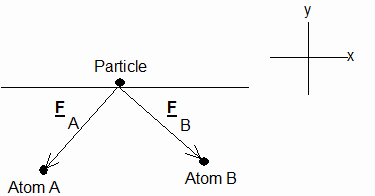Earth Atoms Attracting Surface Particle

It’s clear in Fig. 20.1 that the x-components of FA and FB cancel, and the y-components add. The same is true for every diametrically opposed pair of Earth atoms. Thus we expect the resultant force on the particle to be toward the Earth’s center.

At first glance, calculating the magnitude of this force seems to be considerably complicated by the fact that the distances between the particle and pairs of Earth atoms varies from zero to the Earth’s diameter. That is a mind-boggling lot of forces to add up! But Newton derived a wonderfully simple result: when the force is inversely proportional to the square of the distance, then the sum of all the forces is the same as what the force would be if all the Earth’s atoms were replaced by a "super" particle at the Earth’s center (said "super" particle’s mass being that of the entire Earth).

According to Newton’s third law, we can turn the situation around: the summed forces on all the Earth’s atoms, exerted by the particle, is the same as the force that the particle would exert on a "super" particle at the Earth’s center.

Now picture two spherical masses, in close proximity. Every atom in sphere A attracts every atom in sphere B. What a daunting prospect! But thanks to their spherical shapes, the sum of all the attractive forces is the same as the single attractive force between two "super" particles at the respective spherical centers!

Small wonder the ancient Greeks called the sphere the most perfect of all geometric forms. Newton himself discovered that a sphere can be adequately represented as a particle in many cases. Here is another interesting fact: given N gravitationally attracting atoms (each exclusively occupying some small volume of space), the arrangement that lets the atoms get as mutually close to one another as possible is a sphere. Given a volume of matter, the smallest surface area that can enclose that volume is a spherical surface. That is why all the stars and all the planets (which were fluid at their births) are spheres!

Chapter 21. Force Couples and Torques

Fig. 21.1 depicts a box, subjected to two equal and oppositely directed forces. The total force exerted on the box is zero. Thus according to F=ma the box’s center of mass does not accelerate. But since the two forces do not act along a common line, something happens. The box begins to spin. The combined effect of F1 and F2 is called a torque (for ‘twisting’).

Figure 21.1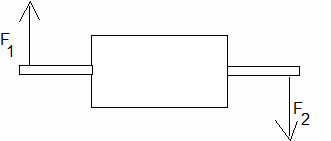A Box Subjected to a Torque

Since acm=0, the velocity of the box’s center of mass is unchanged by the applied torque. But the speeds of other parts of the box are increased by the torque. Thus despite the fact that the box’s center of mass remains at rest, the box as a whole gains kinetic energy.

There are important parallels between F=ma and the equations relating torques to the rates at which the spins of objects change. Indeed just as a macroscopic body can have linear momentum, as a consequence of the motion of its center of mass along some line, so it can have angular momentum as a consequence of rotation of its parts about some axis. The direction of the angular momentum can be found by curling the fingers of the right hand around in the direction of spin. The thumb will then point in the direction of the angular momentum. Similarly, this ‘right hand rule’ can be used to determine the direction of the applied torque. (In Fig. 21.1 the torque points into the page.)

It is customary to denote the angular momentum as L and the torque as t. And just as Fresultant=dpcm/dt, it can also be said that t=dL/dt

One of the world’s most fascinating toys is a gyroscope. Fig. 21.2 schematically depicts a typical gyro. Application of the right hand rule indicates that its angular momentum, L, points in the positive y-direction.

Figure 21.2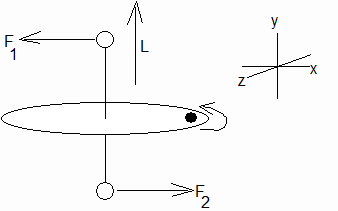A Gyroscope

Now here is an interesting question: what effect will the indicated force couple have? Intuitively it may seem that the gyroscope should rotate counterclockwise around the z-axis. But the torque, created by forces F1 and F2 points out of the page (in the positive z-direction). Thus the torque and angular momentum vector are at right angles. According to t=dL/dt, this torque should cause L to start rotating around the x-axis!

Let us see if we can understand this weird behavior. Consider the particle indicated by a dot near the gyroscope’s rim. In the absence of any torque, this particle travels a circular path in the xz-plane. It does this because the material between the particle and the gyro axis exerts a radial force inward on the particle. And of course the particle exerts an outward force on that material. The magnitude of these action and reaction forces increases with the spin rate of the gyro. Indeed if the spin rate becomes too great, the gyro will fly apart.

Now owing to the gyro’s rigidity, the effect of the force couplet in the figure is to cause a second force to be exerted on the particle. This force, at the moment shown, acts in the positive y-direction. Under the influence of the radial inward force and the upward force, the particle not only circles around the axle, but by the time it’s gone a quarter turn around, it will have risen slightly above the xz plane. This process continues as long as the force couplet is applied to the ends of the axle. In reality the gyro axis rotates around the x-axis when the indicated torque is applied! The motion is called precession.

Note that since F1 and F2 each act at right angles to the motions of the axle’s ends, they do zero work for as long as they cause the gyro to precess. Thus there’s no change in the gyro’s spin rate or rotational kinetic energy. As soon as the torque is removed, the precession halts.

Many devices depend upon gyroscopic behavior. A child’s spinning top does not fall over as a result of it. And we are able to keep ourselves upright on a moving bicycle by turning the front wheel to the right or left. In general gyros maintain their orientation in inertial space when subjected to no torques. Thus a good variation on Newton’s first law would be: When subjected to no torques, a gyroscope maintains its orientation relative to the axes of all inertial frames of reference. This fundamental behavior of gyroscopes makes them useful devices in aerospace navigation. Assuming a gyro can be suspended without friction in a case, the gyro will maintain its orientation relative to the ‘fixed’ stars, regardless of how the airplane or spacecraft rolls, yaws and pitches.

Just as there are parallels between F=ma and rotational mechanics, so does rotation have its own conservation laws. That is, angular momentum and rotational kinetic energy are conserved, quite as linear momentum and energy are. These laws stem from the corollary to Newton’s third law, that for every torque applied by some agent to an object (or system), an equal and oppositely directed torque is applied by the object on the agent.

Chapter 22. Heat.

As scientists explored the many ramifications of Newton’s laws, a second movement was underway investigating the phenomena of thermodynamics (notably heat). In general any object (like the gyroscope in Chap. 21) or any volume of gas contains heat energy. Heat is now known to be a form of kinetic energy. Even though an object’s center of mass is at rest, it has internal kinetic energy. Indeed even though its total momentum (both linear and angular) is zero, it has kinetic energy. How can this be?

The answer became evident with the discovery of atoms. Every macroscopic (big enough to see) object is composed of many atoms. And at temperatures above absolute zero these atoms are not at rest. In a solid, each atom vibrates around some nominal center point (as if connected to its neighbors by several microscopic springs). And in the case of a gas, the atoms dart about, rebounding elastically off other gas atoms (or molecules).

Of course the motions (or momenta) are in all different directions, and the total momentum is essentially zero. Yet there is kinetic energy.

Historically it wasn’t at first obvious that macroscopic objects are composed of myriad atoms. Temperature was used as a measure of heat energy. For a time there was Newtonian mechanics, and there was the evidently different phenomenon of heat flow from hotter to colder objects, etc.

With the discovery of atoms, however, it soon became evident that heat energy is in fact internal kinetic energy, and temperature is proportional to the average atomic/molecular kinetic energy in a substance or gas.

Of course scientists couldn’t observe individual atoms, even in a gas (not to mention a solid). The connections between Newtonian mechanics and thermodynamics had to largely be inferred, using the methods of Statistical Mechanics. Many new insights about the physical world grew out of these studies. It is a fascinating story, and possibly one that you will not gain much exposure to in an undergraduate physics course. But there are many excellent books on the subject, any one of which would make a fascinating read during vacation.

To the eye, a solid’s surface may appear to be placid. But microscopically the surface atoms are darting out and in. Newton’s laws of mechanics can be used to show that heat energy flows from a hotter to a cooler object when their surfaces are brought in contact.

Other phenomena and laws (e.g. Boyle’s law) were seen to be natural consequences of Newton’s laws once atoms had been discovered. It is safe to say that these three laws, coupled with the knowledge that atoms are the fundamental building blocks of nature, paved the way for one of the greatest theoretical syntheses in human history.

Chapter 23. Fluid Dynamics.

Another area of study that initially paralleled Newtonian mechanics was the study of fluid behaviors (moving air, water, etc.) A matter of particular interest was the pressure (or force per unit area) exerted by a fluid on a material surface. Before the discovery of atoms it was known that the greater the flow rate of a fluid over a material surface, the less pressure the fluid exerts on the surface. But the underlying reasons for this phenomenon were unclear.

Fig. 23.1 depicts the flow of a fluid over the top and bottom surfaces of an object. Let us assume for openers that the fluid is incompressible. Then two elements of fluid starting from the leading edge (one going over the top surfaces and the other going over the bottom surfaces) must nominally meet up again at the trailing edge.

Figure 23.1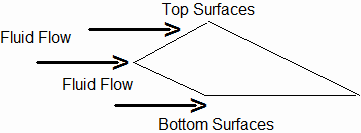Fluid Flowing over Top and Bottom Surfaces

Now clearly the distance over the top surfaces, from leading to trailing edge, is greater than the distance over the bottom surfaces. Thus the fluid must travel faster over the top than over the bottom if everything is to meet up again at the trailing edge. The pressure on the top is accordingly less than the pressure on the bottom. Part of this difference is offset by the greater surface area of the top surface. But the offset is not complete. There is a net force upward on the object.

Of course we can as well view things from the fluid’s rest frame. In this case the object moves through the fluid. The net force upward is still there.

Let us now replace the incompressible fluid in Fig. 23.1 with air (or any gas). Gases are compressible and, assuming the temperature is above absolute zero, the molecules in the air’s "rest frame" are darting about in all directions.

Air molecules encountering the object’s leading surfaces will rebound from them. But the net force on these two surfaces is zero. In effect the air molecules will be "swept" upward and downward. Since the object’s trailing bottom edge is parallel to the air flow, this sweeping action has negligible effect on the number of molecules rebounding from it every second. But the top trailing surface is not parallel to the air flow. As a consequence, the sweeping action of the top leading surface casts a "shadow" above the top trailing surface … a partial vacuum is created above the top trailing surface. If the flow is fast enough, the thermal motion of air above the object cannot fill this vacuum in. Many of the air molecule/surface collisions that would have occurred, if the air flow rate was zero, do not now occur. The result is that a net upward force is exerted on the trailing surfaces of the object. In aeronautical terms, the object (which is of course a wing) experiences lift. It will defy gravity, and will even climb. We need only switch frames, into the air’s nominal rest frame, with the wing being pushed through the air (by a jet, propeller or what not). The lift is still there. If we attach a couple of these wings to a tube (fuselage) and add a few other control surfaces, we have a plane!

The lift on the trailing surfaces of the wing can ultimately be attributed to the rarefaction (or partial vacuum) created above the wing’s upper trailing surface. It is contingent upon the air not backfilling (due to thermal motion) before the trailing part of the wing has passed out of the rarefaction zone. If the temperature is too high … if the mean air molecular speed is too great … partial backfilling will occur before the trailing edge of the wing passes out of the rarefaction zone … the wing will start losing its lift. Thus the lift effect works best in cool air. Indeed in places where it gets really hot in the summertime (e.g. Arizona), airport authorities may sometimes deem it advisable to prohibit takeoffs and landings. There won’t be enough lift on airplane wings, at normal takeoff and landing speeds, to provide adequate climb and controlled descent.

Chapter 24. Collisions.

We can define a gas (and a liquid) to be a collection of particles that aren’t bound to one another. (A solid would correspondingly be a collection of particles that are bound to one another.) In an ideal gas the particles are geometric points and, statistically speaking, collisions don’t occur. In a real gas each atom or molecule occupies a small but finite volume of space. Depending on the density of the gas, collisions occur more or less frequently. Any given collision may range from a grazing brush between two atoms/molecules to a head-on crash.

A collision between two particles is defined to be elastic when the total kinetic energy after the collision equals the total kinetic energy before the collision. "Total kinetic energy" in this case refers to mv2/2, and does not include heat (or "internal" particle kinetic energy). The individual particle kinetic energies may change as a consequence of the collision. But the total kinetic energy remains constant.

A macroscopic example of near-perfect elastic collisions is found in billiards or pool. Pool balls are by design very hard, and they rebound elastically off one another. A microscopic example of elastic collisions is found in gases.

Inelastic collisions are defined to be those where the total kinetic energy after the collision is not equal to the total kinetic energy before. Typically the total kinetic energy after the collision is less than the total kinetic energy before. An example is when a bullet is shot into a block of wood.

What happens to the lost kinetic energy when a collision is inelastic? For the most part it may be converted to heat (or internal kinetic) energy. When a bullet is fired into a block of wood the total heat energy, of bullet and block, is less before the collision than after. Indeed the gain in total heat energy practically equals the loss in total kinetic energy. (There will be other forms of energy also created, but they are often considered to be negligible. For example, in air there will be a loud "whack" … acoustic energy will be generated.)

Now it is a useful fact that the total momentum remains unchanged in all types of collisions. And together, the Conservation of Momentum and Energy laws can be very useful in analyzing and predicting the outcomes of collisions. This is true for collisions between all manner of particles. It is a fact worth remembering. If you go on to study quantum physics, you’ll learn that light interacts with material particles in a particulate fashion. And the "collision rules" when a "light particle" (or a photon) collides with a material particle (such as an electron) are essentially the same as when two material particles collide with one another. Interestingly enough, however, photons don’t seem to collide with one another (as electrons do).

Chapter 25. Periodic Motions.

One of the more interesting toys that were in vogue for a time were "Super Balls." These are hard rubber balls which rebound almost elastically from a hard floor. That is, you can drop a super ball onto a hard floor, and the ball will almost bounce all the way back up to your hand. The collisions of ball and floor are nearly elastic.

Of course there are never truly perfect elastic collisions between macroscopic objects. And the super ball doesn’t quite bounce all the way back up to the hand that dropped it. It (and the floor) heat up slightly with each bounce, and again acoustic energy is created. If the collision between ball and floor were perfectly elastic, the ball would continue bouncing up to the same height repeatedly, forever.

Motions that are repeated in time are said to be periodic. The time from the arbitrarily defined start of one cycle to the start of the next is called the motion’s period, and is often symbolized as t. The motion is periodic if the velocity at time (t+t) is the same as the velocity at time t.

The frequency of a periodic motion is defined to be the reciprocal of the period. It is often symbolized as n. Thus n=1/t. The frequency is therefore the number of cycles completed in unit time (e.g. cycles per second). Note that "cycles" is considered to be dimensionless. When the unit of time is the second, then frequencies are expressed in Hertz (or Hz): 10 cycles/second is expressed as 10 Hz or 10 sec-1.

An ideal super ball, bouncing off a hard surface, would be an example of a periodic motion. If the time required for the ball to start from a state of rest, accelerate downward until it rebounds from the floor, and decelerate upward to a state of rest again is t, then at any moment t+t its velocity will be the same as at time t. Many periodic motions (like the bouncing super ball) are symmetric, and v(t+t/2) = -v(t).

Another important example of periodic motion is that of a particle oscillating on an ideal, anchored spring. In this case the motion is sinusoidal … the parameters vary sinusoidally with time. For example, if "A" is the maximum distance the spring is stretched (and/or compressed), and if the particle oscillates about the origin (where the spring is relaxed) along the x-axis, then x = A sin(wt), where w=2pn. And in such cases vx = wA cos(wt), etc.

"w" is called the angular frequency for the following reason. Imagine a particle circling the origin of a rectangular coordinate system, in a counterclockwise direction and at constant speed v and in the xy-plane. Fig. 25.1 illustrates.

Figure 25.1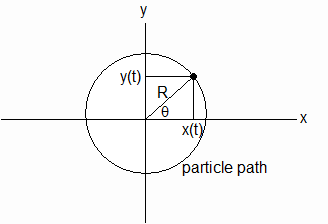Particle in Circular Orbit

For this type of motion, x(t)=Rcos(q) and y(t)=Rsin(q). Let us say that the particle is at x=R, y=0 at time t=0. Then q=wt, where q is expressed in radians and w is expressed in radians per second. (2p radians equals 360 degrees. Like cycles, radians are dimensionless … the units of w are second-1.) Thus w is the rate of increasing angle q, and hence is called the angular frequency.

Sinusoidal motions are of great interest in physics, since they occur (or are closely approximated) in many situations. For example, the motions of a solid’s atoms, about their nominal center points, are practically sinusoidal over a broad range of temperatures.

Mathematically it is a useful fact that the x- and y-position components of a particle, circling the origin at constant speed, are the same as those of two particles, one oscillating on the x-axis and the other on the y-axis. But physically the two phenomena are not equivalent. For example, the electromagnetic field of a charge going in a circle is not the same as two half charges oscillating along the x- and y-axes respectively. (This is obvious when w approaches zero.)

Chapter 26. Waves.

Imagine that we have a very long wire, anchored at one end to a rigid wall and with Leroy’s hand holding the other end. Fig. 26.1 illustrates.

Figure 26.1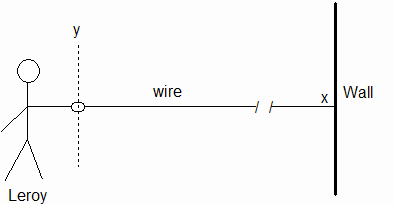Leroy and the Wire

At time t=0 Leroy begins shaking his hand up and down such that the position of his hand is y = A sin(wt). What happens? As you might know from experience, the wire begins to undulate up and down, not only at Leroy’s hand, but progressively outward toward the wall. The speed at which these undulations propagate away from Leroy will depend, in part, on how tightly Leroy pulls the wire in the negative x-direction (i.e., the speed will depend, in part, on the tension that Leroy maintains in the wire.)

If we observe the wire at some point x>0, between Leroy and the wall (and before the undulations have reached the wall), we find that y(x,t) = A sin(wt-vt), where v is the speed at which the undulations move away from Leroy’s hand and toward the wall. In other words, the vertical acceleration that Leroy imparts at x=0 is reproduced at point x>0 and at time t>0 according to x = vt.

Although any small section of the wire, at any given x>0, is moving up and down, our eyes discern a wave traveling down the wire in the positive x-direction. This wave looks like a sine curve, moving to the right. The speed of the movement to the right is the wave’s speed, and the distance between any two values of x, where the curve completes one complete sine curve, is called the wavelength, l. Evidently the wave’s speed equals the wavelength divided by the period (or multiplied by the frequency): v = l/t = ln.

Waves of all kinds are encountered in the physical world. The space we occupy is constantly flooded with a staggering mix of electromagnetic waves. We need only turn on a radio or television to sample them. (Or, where there is light, we need only open our eyes.)

Waves like those in the wire, where the oscillations occur perpendicular to the direction of wave propagation, are referred to as transverse waves. When the oscillation occurs back and forth along the line of propagation, the waves are said to be longitudinal waves. An example of a longitudinal wave is a sound (or acoustic) wave. With sound, air molecules oscillate back and forth along the line of propagation. A stereo speaker’s diaphragm oscillates back and forth, compressing and rarefying the air in front of it. A longitudinal compression/rarefaction wave propagates away. A fraction of a second later the energy (and momentum) in a portion of this wave reaches our eardrums, causing them to reproduce the pattern of speaker diaphragm oscillations. These eardrum oscillations cause nerve impulses to be transmitted to the part of our brain that interprets acoustic stimuli, and (with luck) we hear beautiful music!

In order to understand electromagnetic waves (light, radio, TV, …) we must use Maxwell’s equations. But Newton’s laws can be used to analyze the waves in taut wires, sound waves, and a host of other wave types.

In the case of a taut wire (Fig. 26.1) Leroy stretches the wire closest to his hand when he lifts/lowers his hand above/below y=0. (i.e., the shortest distance between two points is a straight line. Thus …) In trying to return to an unstretched state, parts of the wire accelerate upward or downward. Here again, kinetic and potential energy ebb back and forth. And here again the overall (or "global") wire behavior can be understood by applying Newton’s laws to the parts (in this case, to tiny wire segments).

Chapter 27. Interference

One of the author’s more memorable experiences occurred one day when a physics professor turned a low frequency sound generator on in a large lecture hall. The acoustic wave from the sound generator quickly spread out to the walls, floor and ceiling, and reflections generated a host of additional waves. In a fraction of a second the hall was filled with sound waves traveling in every imaginable direction. Like all waves, these sound waves freely passed through one another. (i.e., they readily occupied a common space.)

The fascinating thing about this experiment was the nature of the waves. We were instructed by the professor to slowly move around in the lecture hall. And when we did so, we made an astounding discovery. In one place we could hear a loud, low frequency hum. It was so loud that it was all but impossible to make out what other students were saying. Yet only a step or two away we heard no hum at all, and could easily hear what other students were saying.

Some years later the author saw a similar experiment performed with an electromagnetic wave generator and a large (reflecting) metal sheet. The professor turned the generator on and held a rod antenna between generator and sheet. The ends of the antenna were wired to a small bulb, and the electromagnetic signal was powerful enough to make the bulb glow. Yet when the antenna was moved farther away from the generator, the bulb went out. It was as if no wave existed at the second location. Further displacement toward the reflecting sheet caused the bulb to glow again!

What lay at the base of these two phenomena were standing waves (acoustic and electromagnetic). Standing waves owe their existence to the ability of two or more waves to pass through a common point in space. When this occurs, the undulations of the thing(s) that oscillate (air molecules, electric field vectors, etc.) add to produce single, resultant undulations. The interesting thing is that, when two waves propagate in opposite directions along a common line, nodes and anti-nodes may be created. A node is where the waves sum to produce a null effect. And an anti-node is where they sum to produce a maximum undulation.

This adding behavior of waves is known as interference. Where the undulations reinforce each other, the interference is said to be constructive. (And where the undulations oppose each other the interference is said to be destructive.)

In mechanics, forces add to produce resultant forces. (We have already investigated that in a previous chapter.) In general, when vector quantities add (as forces, electromagnetic field vectors, etc. do) the quantities are said to obey the Principle of Superposition.

In Fig. 26.1 Leroy can (with the wall’s help) set up standing waves. The propagating waves, initially created by Leroy, will eventually reach the wall and a reflected wave will be sent back toward Leroy. If Leroy wiggles his end of the wire up and down at the right frequency, a standing wave will result. The wire will arc up and down between two or more nodes. There will still be wave action, but the resultant wave will seem to propagate neither to the right nor to the left ... it will "stand still" (hence the name "standing wave").

One of the requirements for creating a standing wave is that Leroy wiggle his end of the wire at the right frequency. A standing wave presumes that an integral number of half wavelengths exist when both ends of an oscillating wire (for example) are anchored. Frequencies meeting this requirement are said to be "resonant" frequencies. They play an important role not only in "classical" phenomena (those explainable by Newtonian and Maxwellian theory), but also in wave mechanics (or quantum theory).

At first (after Newton but before the discovery of atoms) many waves were not understood to be a consequence of Newton’s laws. But with the discovery of atoms it became possible to analyze such waves in terms of Newtonian mechanics. The notable exceptions were electromagnetic waves. One must use Maxwell’s equations to understand them. Indeed Maxwell was the first to realize that light is an electromagnetic wave! His announcement sent shock waves throughout the scientific community.

Concluding Remarks.

If ever there were two inspired moments in human history, it was when Newton wrote his three laws of mechanics, and when Maxwell and Lorentz worked out the electromagnetic force law. For all of these laws work in every inertial frame of reference and are relativistically correct.

Equally as marvelous, Newton’s laws and the Maxwell-Lorentz force law are connected in some very fundamental ways. Part of the force exerted on a naked charge … usually the dominant part … equates to d(memv)/dt. (The other, less dominant part causes radiation.) And the reaction force in Newton’s third law is seen to be nothing more nor less than an acceleration-induced electric force when the fields of an accelerated charge are solved for.

Einstein’s Special Theory of Relativity wrought profound changes to Newtonian mechanics. Many of the cherished, "axiomatic" assumptions of the pre-relativity era fell. Mass, force and acceleration were no longer matters of agreement among inertial frames. Indeed inertial observers even disagree about the lengths of solid objects and the rates at which clocks run. It is a fascinating subject, fully worthy of its own book.

Despite such revolutionary (and sometimes counter-intuitive) changes, Newton’s three laws come shining through (as do Maxwell’s equations and Lorentz’s force law). F=d(mv)/dt is relativistically correct in every inertial frame of reference. But, owing to the dependence of mass on particle speed (which of course varies from inertial frame to inertial frame), F=ma is not valid in relativity.

How did Newton know to write his second law in the relativistically correct form? Was it simply fortuitous? Or did he have something going for him that we can only speculate about? And did he understand that his third law contained the seeds of the great conservation laws?

With regard to his Universal Law of Gravitation, no mention of time is made. But today it is believed that gravitational interactions, like electromagnetic interactions, pass between material objects at a finite speed (namely the speed of light). Does an "uncharged" body generate some sort of gravitational waves when forced to oscillate, as a charged body does? General Relativity theory suggests that it does. The search is on to detect such waves!

It is an intriguing fact that Maxwell’s field equations (and the Lorentz force law), coupled with Newton’s second law, can be used to demonstrate that a moving "atom" is actually length-contracted, and that temporal processes occur more slowly in moving systems (a phenomenon referred to as "time dilation"). Thus the seeds of some of the most counter-intuitive aspects of Special Relativity lay waiting to be derived by applying Newton’s laws and the theory of Maxwell/Lorentz.

As these words are written, it has been discovered that even uncharged elementary particles (e.g. neutrons) are composed of charged particles (quarks). The possibility that "neutral matter" may, in the final analysis, be only a figment of our imaginations raises some intriguing questions. Might we find that the "inertial," equal and oppositely directed reaction forces of Newton’s third law, in the case of "uncharged" atoms, neutrons, etc., are ultimately electric forces when we "peel back the veil," and peer within?

All in all, mechanics in one form or another continues to be one of the most interesting branches of physics. Observing things moving around in our personal spaces, and wanting to predict those motions, seems to be rooted in our very genes. Many ingenious recipes for making such predictions have been suggested through the years. But none have enjoyed greater staying power than Isaac Newton’s incredible laws!